### Home > CALC > Chapter 11 > Lesson 11.4.1 > Problem11-131

11-131.
1.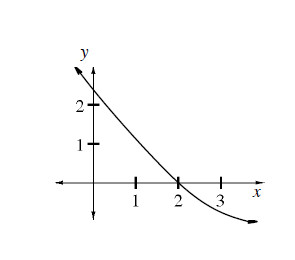Multiple Choice: f(x), shown below, is twice differentiable. Examine the graph and decide which statement below is true. Homework Help ✎

1.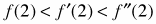2.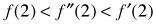3.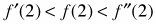4.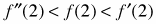5.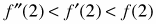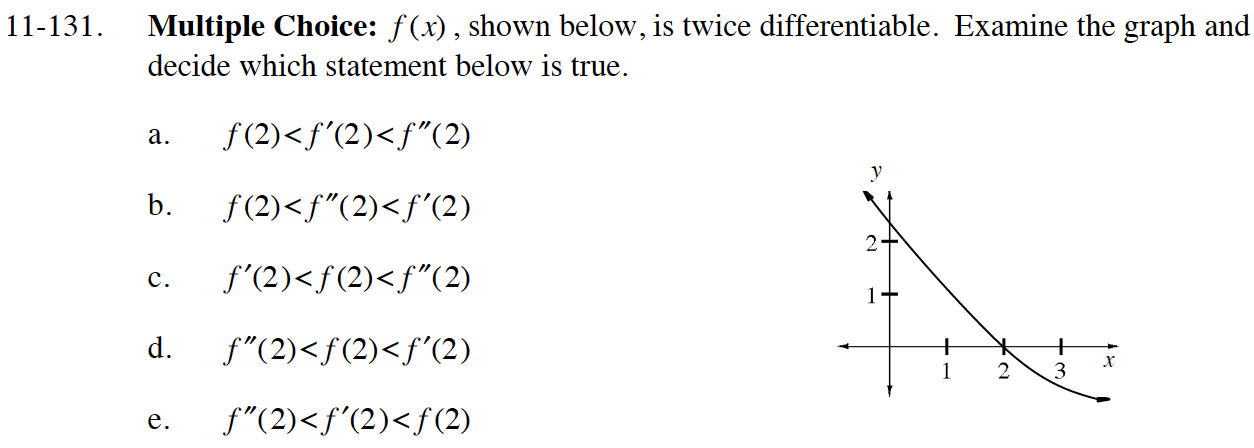f(2) = 0
f′(2) = slope at x = 2 (positive or negative?)
f″(2) = concavity at x = 2 (up or down?)